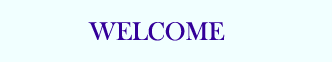TUTORIALS

# ASP - AJAX and ASP

AJAX is used to create more interactive applications.

## AJAX ASP Example

The following example will demonstrate how a web page can communicate with a web server while a user type characters in an input field:

## Example

 Start typing a name in the input field below: First name: Suggestions:

View it »

## Example Explained - The HTML Page

When a user types a character in the input field above, the function "showHint()" is executed. The function is triggered by the "onkeyup" event:



Start typing a name in the input field below:

First name:

Suggestions:

Source code explanation:

If the input field is empty (str.length==0), the function clears the content of the txtHint placeholder and exits the function.

If the input field is not empty, the showHint() function executes the following:

• Create an XMLHttpRequest object
• Create the function to be executed when the server response is ready
• Send the request off to a file on the server
• Notice that a parameter (q) is added to the URL (with the content of the input field)

## The ASP File

The page on the server called by the JavaScript above is an ASP file called "gethint.asp".

The source code in "gethint.asp" checks an array of names, and returns the corresponding name(s) to the browser:

 <% response.expires=-1 dim a(30) 'Fill up array with names a(1)="Anna" a(2)="Brittany" a(3)="Cinderella" a(4)="Diana" a(5)="Eva" a(6)="Fiona" a(7)="Gunda" a(8)="Hege" a(9)="Inga" a(10)="Johanna" a(11)="Kitty" a(12)="Linda" a(13)="Nina" a(14)="Ophelia" a(15)="Petunia" a(16)="Amanda" a(17)="Raquel" a(18)="Cindy" a(19)="Doris" a(20)="Eve" a(21)="Evita" a(22)="Sunniva" a(23)="Tove" a(24)="Unni" a(25)="Violet" a(26)="Liza" a(27)="Elizabeth" a(28)="Ellen" a(29)="Wenche" a(30)="Vicky" 'get the q parameter from URL q=ucase(request.querystring("q")) 'lookup all hints from array if length of q>0 if len(q)>0 then   hint=""   for i=1 to 30     if q=ucase(mid(a(i),1,len(q))) then       if hint="" then         hint=a(i)       else         hint=hint & " , " & a(i)       end if     end if   next end if 'Output "no suggestion" if no hint were found 'or output the correct values if hint="" then   response.write("no suggestion") else   response.write(hint) end if %>

Explanation: If there is any text sent from the JavaScript (strlen(\$q) > 0), the following happens:

1. Find a name matching the characters sent from the JavaScript
2. If no match were found, set the response string to "no suggestion"
3. If one or more matching names were found, set the response string to all these names
4. The response is sent to the "txtHint" placeholder